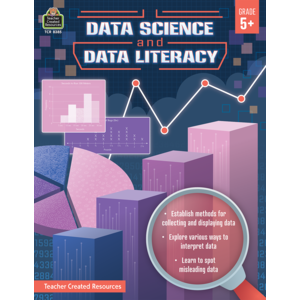## Common Core State Standards (CCSS) Correlations### Data Science and Data Literacy Gr. 5

• 5th and up
• 80 pages
• Product ID: TCR8385

## Mathematics

### Domain - Measurement and Data, Grade 3

Represent and interpret data.

Math.3.MD.B.3: Draw a scaled picture graph and a scaled bar graph to represent a data set with several categories. Solve one- and two-step “how many more” and “how many less” problems using information presented in scaled bar graphs. For example, draw a bar graph in which each square in the bar graph might represent 5 pets.

Activity Pages
Bar Graphs 7-16
Pie Charts 17-26

### Domain - Measurement and Data, Grade 4

Represent and interpret data.

Math.4.MD.B.4: Make a line plot to display a data set of measurements in fractions of a unit (1/2, 1/4, 1/8). Solve problems involving addition and subtraction of fractions by using information presented in line plots. For example, from a line plot find and interpret the difference in length between the longest and shortest specimens in an insect collection.

Activity Pages
Line Plots 27-36
Dot Plots 37-46

Geometric measurement: understand concepts of angle and measure angles.

Math.4.MD.C.5: Recognize angles as geometric shapes that are formed wherever two rays share a common endpoint, and understand concepts of angle measurement:

Activity Pages
Pie Charts 17-26

### Domain - Number and Operations-Fractions, Grade 5

Use equivalent fractions as a strategy to add and subtract fractions.

Math.5.NF.A.2: Solve word problems involving addition and subtraction of fractions referring to the same whole, including cases of unlike denominators, e.g., by using visual fraction models or equations to represent the problem. Use benchmark fractions and number sense of fractions to estimate mentally and assess the reasonableness of answers. For example, recognize an incorrect result 2/5 + 1/2 = 3/7, by observing that 3/7 < 1/2.

Activity Pages
Line Plots 27-36
Dot Plots 37-46

### Domain - Measurement and Data, Grade 5

Represent and interpret data.

Math.5.MD.B.2: Make a line plot to display a data set of measurements in fractions of a unit (1/2, 1/4, 1/8). Use operations on fractions for this grade to solve problems involving information presented in line plots. For example, given different measurements of liquid in identical beakers, find the amount of liquid each beaker would contain if the total amount in all the beakers were redistributed equally.

Activity Pages
Bar Graphs 7-16
Pie Charts 17-26
Line Plots 27-36
Histograms 47-56

### Domain - Understand ratio concepts and use ratio reasoning to solve problems., Grade 6

Use ratio and rate reasoning to solve real-world and mathematical problems, e.g., by reasoning about tables of equivalent ratios, tape diagrams, double number line diagrams, or equations.

Math.6.RP.A.3c: Find a percent of a quantity as a rate per 100 (e.g., 30% of a quantity means 30/100 times the quantity); solve problems involving finding the whole, given a part and the percent.

Activity Pages
Pie Charts 17-26

### Domain - Statistics and Probability, Grade 6

Develop understanding of statistical variability.

Math.6.SP.A.2: Understand that a set of data collected to answer a statistical question has a distribution which can be described by its center, spread, and overall shape.

Activity Pages
Bar Graphs 7-16
Pie Charts 17-26
Line Plots 27-36
Dot Plots 37-46
Histograms 47-56
Box Plots 57-66

Develop understanding of statistical variability.

Math.6.SP.A.3: Recognize that a measure of center for a numerical data set summarizes all of its values with a single number, while a measure of variation describes how its values vary with a single number.

Activity Pages
Line Plots 27-36
Dot Plots 37-46
Histograms 47-56
Box Plots 57-66

Summarize and describe distributions.

Math.6.SP.B.4: Display numerical data in plots on a number line, including dot plots, histograms, and box plots.

Activity Pages
Bar Graphs 7-16
Pie Charts 17-26
Line Plots 27-36
Dot Plots 37-46
Histograms 47-56
Box Plots 57-66

### Domain - Summarize and describe distributions., Grade 6

Summarize numerical data sets in relation to their context, such as by:

Math.6.SP.B.5a: Reporting the number of observations.

Activity Pages
Bar Graphs 7-16
Pie Charts 17-26
Line Plots 27-36
Dot Plots 37-46
Histograms 47-56
Box Plots 57-66

Summarize numerical data sets in relation to their context, such as by:

Math.6.SP.B.5c: Giving quantitative measures of center (median and/or mean) and variability (interquartile range and/or mean absolute deviation), as well as describing any overall pattern and any striking deviations from the overall pattern with reference to the context in which the data were gathered.

Activity Pages
Line Plots 27-36
Dot Plots 37-46
Histograms 47-56
Box Plots 57-66

### Domain - Statistics and Probability, Grade 7

Use random sampling to draw inferences about a population.

Math.7.SP.A.1: Understand that statistics can be used to gain information about a population by examining a sample of the population; generalizations about a population from a sample are valid only if the sample is representative of that population. Understand that random sampling tends to produce representative samples and support valid inferences.

Activity Pages
Bar Graphs 7-16
Pie Charts 17-26
Line Plots 27-36
Dot Plots 37-46
Histograms 47-56
Box Plots 57-66

Use random sampling to draw inferences about a population.

Math.7.SP.A.2: Use data from a random sample to draw inferences about a population with an unknown characteristic of interest. Generate multiple samples (or simulated samples) of the same size to gauge the variation in estimates or predictions. For example, estimate the mean word length in a book by randomly sampling words from the book; predict the winner of a school election based on randomly sampled survey data. Gauge how far off the estimate or prediction might be.

Activity Pages
Line Plots 27-36
Dot Plots 37-46
Histograms 47-56
Box Plots 57-66